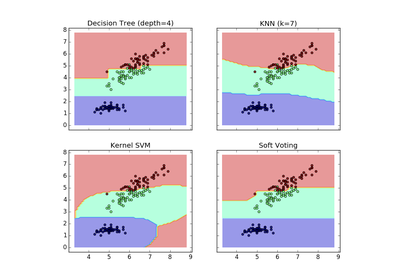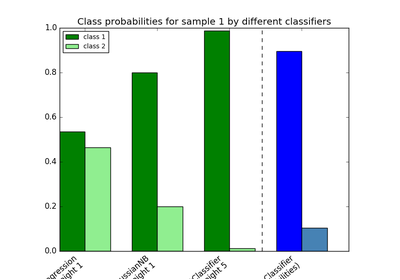# `sklearn.ensemble`.VotingClassifier¶

class `sklearn.ensemble.``VotingClassifier`(estimators, voting='hard', weights=None, n_jobs=1)[source]

Soft Voting/Majority Rule classifier for unfitted estimators.

New in version 0.17.

Read more in the User Guide.

Parameters: estimators : list of (string, estimator) tuples Invoking the `fit` method on the `VotingClassifier` will fit clones of those original estimators that will be stored in the class attribute self.estimators_. voting : str, {‘hard’, ‘soft’} (default=’hard’) If ‘hard’, uses predicted class labels for majority rule voting. Else if ‘soft’, predicts the class label based on the argmax of the sums of the predicted probabilities, which is recommended for an ensemble of well-calibrated classifiers. weights : array-like, shape = [n_classifiers], optional (default=`None`) Sequence of weights (float or int) to weight the occurrences of predicted class labels (hard voting) or class probabilities before averaging (soft voting). Uses uniform weights if None. n_jobs : int, optional (default=1) The number of jobs to run in parallel for `fit`. If -1, then the number of jobs is set to the number of cores. estimators_ : list of classifiers The collection of fitted sub-estimators. classes_ : array-like, shape = [n_predictions] The classes labels.

Examples

```>>> import numpy as np
>>> from sklearn.linear_model import LogisticRegression
>>> from sklearn.naive_bayes import GaussianNB
>>> from sklearn.ensemble import RandomForestClassifier, VotingClassifier
>>> clf1 = LogisticRegression(random_state=1)
>>> clf2 = RandomForestClassifier(random_state=1)
>>> clf3 = GaussianNB()
>>> X = np.array([[-1, -1], [-2, -1], [-3, -2], [1, 1], [2, 1], [3, 2]])
>>> y = np.array([1, 1, 1, 2, 2, 2])
>>> eclf1 = VotingClassifier(estimators=[
...         ('lr', clf1), ('rf', clf2), ('gnb', clf3)], voting='hard')
>>> eclf1 = eclf1.fit(X, y)
>>> print(eclf1.predict(X))
[1 1 1 2 2 2]
>>> eclf2 = VotingClassifier(estimators=[
...         ('lr', clf1), ('rf', clf2), ('gnb', clf3)],
...         voting='soft')
>>> eclf2 = eclf2.fit(X, y)
>>> print(eclf2.predict(X))
[1 1 1 2 2 2]
>>> eclf3 = VotingClassifier(estimators=[
...        ('lr', clf1), ('rf', clf2), ('gnb', clf3)],
...        voting='soft', weights=[2,1,1])
>>> eclf3 = eclf3.fit(X, y)
>>> print(eclf3.predict(X))
[1 1 1 2 2 2]
>>>
```

Methods

 `fit`(X, y[, sample_weight]) Fit the estimators. `fit_transform`(X[, y]) Fit to data, then transform it. `get_params`([deep]) Return estimator parameter names for GridSearch support `predict`(X) Predict class labels for X. `score`(X, y[, sample_weight]) Returns the mean accuracy on the given test data and labels. `set_params`(\*\*params) Set the parameters of this estimator. `transform`(X) Return class labels or probabilities for X for each estimator.
`__init__`(estimators, voting='hard', weights=None, n_jobs=1)[source]
`fit`(X, y, sample_weight=None)[source]

Fit the estimators.

Parameters: X : {array-like, sparse matrix}, shape = [n_samples, n_features] Training vectors, where n_samples is the number of samples and n_features is the number of features. y : array-like, shape = [n_samples] Target values. sample_weight : array-like, shape = [n_samples] or None Sample weights. If None, then samples are equally weighted. Note that this is supported only if all underlying estimators support sample weights. self : object
`fit_transform`(X, y=None, **fit_params)[source]

Fit to data, then transform it.

Fits transformer to X and y with optional parameters fit_params and returns a transformed version of X.

Parameters: X : numpy array of shape [n_samples, n_features] Training set. y : numpy array of shape [n_samples] Target values. X_new : numpy array of shape [n_samples, n_features_new] Transformed array.
`get_params`(deep=True)[source]

Return estimator parameter names for GridSearch support

`predict`(X)[source]

Predict class labels for X.

Parameters: X : {array-like, sparse matrix}, shape = [n_samples, n_features] Training vectors, where n_samples is the number of samples and n_features is the number of features. maj : array-like, shape = [n_samples] Predicted class labels.
`predict_proba`

Compute probabilities of possible outcomes for samples in X.

Parameters: X : {array-like, sparse matrix}, shape = [n_samples, n_features] Training vectors, where n_samples is the number of samples and n_features is the number of features. avg : array-like, shape = [n_samples, n_classes] Weighted average probability for each class per sample.
`score`(X, y, sample_weight=None)[source]

Returns the mean accuracy on the given test data and labels.

In multi-label classification, this is the subset accuracy which is a harsh metric since you require for each sample that each label set be correctly predicted.

Parameters: X : array-like, shape = (n_samples, n_features) Test samples. y : array-like, shape = (n_samples) or (n_samples, n_outputs) True labels for X. sample_weight : array-like, shape = [n_samples], optional Sample weights. score : float Mean accuracy of self.predict(X) wrt. y.
`set_params`(**params)[source]

Set the parameters of this estimator.

The method works on simple estimators as well as on nested objects (such as pipelines). The latter have parameters of the form `<component>__<parameter>` so that it’s possible to update each component of a nested object.

Returns: self :
`transform`(X)[source]

Return class labels or probabilities for X for each estimator.

Parameters: X : {array-like, sparse matrix}, shape = [n_samples, n_features] Training vectors, where n_samples is the number of samples and n_features is the number of features. If `voting=’soft’`: : array-like = [n_classifiers, n_samples, n_classes] Class probabilities calculated by each classifier. If `voting=’hard’`: : array-like = [n_samples, n_classifiers] Class labels predicted by each classifier.

## Examples using `sklearn.ensemble.VotingClassifier`¶Plot the decision boundaries of a VotingClassifierPlot class probabilities calculated by the VotingClassifier